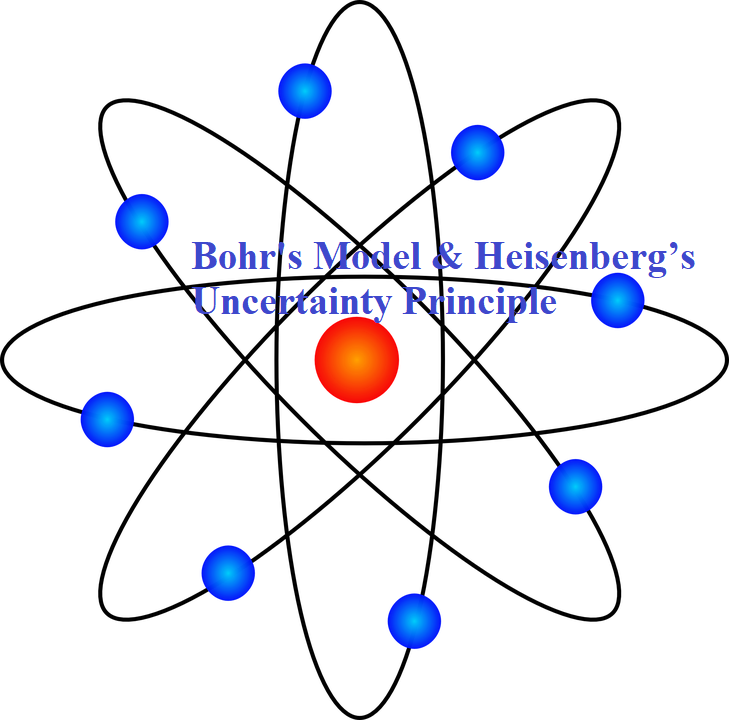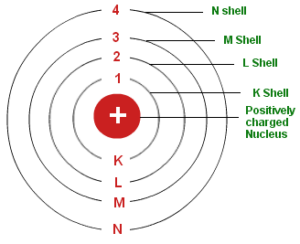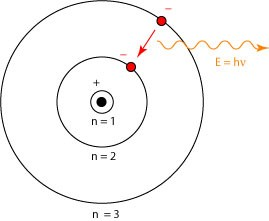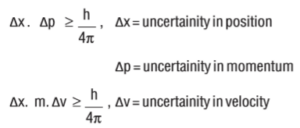# Bohr’s Model & Heisenberg’s Uncertainty Principle## Bohr’s Model & Heisenberg’s Uncertainty Principle

Bohr’s Model & Heisenberg’s Uncertainty Principle

Bohr’s Model of Atom: The general features of the structure of the hydrogen atom and its spectrum was first explained by Niels Bohr. The important postulates of his theory are:

1. The electron in the hydrogen atom can move around the nucleus in circular paths of fixed radius and energy. These paths are called orbits or stationary states or allowed energy states. These energy levels are numbered as 1,2,3 etc or as K, L, M, N, etc. These numbers are known as Principal quantum numbers.2. The energy of an electron in an orbit does not change with time. However, when an electron absorbs energy, it will move away from the nucleus (i.e. to a higher energy level) and when it loses energy, it will move towards the nucleus (i.e. to a lower energy level).3. The radius of orbits can be given by the equation: rn = a0 n2 where a0 = 52.9
Thus, the radius of the first stationary state is 52.9 pm (called the Bohr radius). As n increases, the value of r will increase.
4. The energy of the electron in an orbit is given by the expression: En = -RH (1/n2), where n = 1,2,3…… and RH is a constant called Rydberg constant. Its value is 2.18×10-18. The energy of the lowest state (the ground state) is given by E1 = –2.18×10–18J. As the value of n increases, the energy of the electron also increases.
5. The frequency of radiation absorbed or emitted when the transition occurs between two stationary states that differ in energy by ΔE, is given by: ν = ΔE/h = E2 – E1/h
Where E1 and E2 are the energies of lower and higher energy levels respectively. This expression is commonly known as Bohr’s frequency rule.
6. The angular momentum of an electron is an integral multiple of h/2π. i.e. mevr = nh/2π
Where me is the mass of the electron, v is the velocity of electron and r is the radius of Bohr orbit. n = 1,2,3… Thus an electron can move only in those orbits whose angular momentum is an integral multiple of h/2π. So only certain fixed orbits are allowed.

• Significance of Negative Sign in Energy Expression: When the electron is free from the influence of the nucleus, its energy is taken as zero. In this situation, the electron is at the orbit i.e at infinite distance from the nucleus with n = ∞. When the electron is attracted by the nucleus and is present in orbit n, the energy is emitted and its energy is lowered. That is the reason for the presence of a negative sign in the equation.
• Limitations of Bohr Atom Model: Bohr atom model could explain the stability and line spectra of the hydrogen atom and hydrogen-like ions (e.g. He+, Li2+, Be3+ etc). But it has the following limitations:
1. It could not explain the fine spectrum of the hydrogen atom.
2. It could not explain the spectrum of atoms other than
3. It was unable to explain the splitting of spectral lines in the presence of the electric field (Stark effect) and in the magnetic field (Zeeman effect).
4. It could not explain the ability of atoms to form molecules by chemical bonds.
5. It did not consider the wave character of matter and Heisenberg’s uncertainty principle.
• Dual Behaviour of Matter – de Broglie’s equation: The French physicist Louis de Broglie postulated that like radiation, matter also exhibits dual behaviour i.e., both particle and wave-like properties. The wavelength (l) of the matter-wave is related to the momentum (p) by the equation: λ = h/p  = h/mv                                                                                           Where m = mass, v = velocity and h is Planck’s constant.                                                     The above equation is known as de Broglie’s equation
• Heisenberg’s Uncertainty Principle: According to this law it is totally impossible to measure simultaneously, both the exact position and exact momentum (or velocity) of a moving microscopic particle or body.

Mathematically this law can be represented as:Significance of Uncertainty Principle: Heisenberg’s Uncertainty Principle is significant only for the motion of microscopic objects and is not applicable to macroscopic objects. According to this Principle, we cannot determine the exact position and momentum of an electron. Thus, it rules out the existence of definite paths or orbits of electrons. We can only say the probability of finding an electron at a given point.